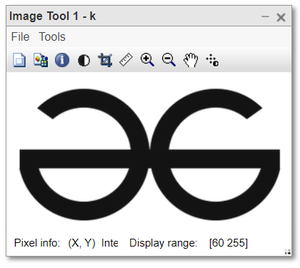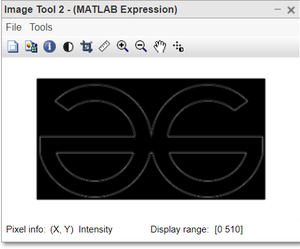Laplacian Filter using Matlab

• Difficulty Level : Expert
• Last Updated : 11 Nov, 2021

Laplacian filter is a second-order derivate filter used in edge detection, in digital image processing. In 1st order derivative filters, we detect the edge along with horizontal and vertical directions separately and then combine both. But using the Laplacian filter we detect the edges in the whole image at once.

The Laplacian Operator/Filter is = [0 1 0;
1 -4 1;
0 1 0]
here the central value of filter is negative.
Or
Filter is = [0 -1 0;
-1 4 -1;
0 -1 0],
here the central value of filter is positive.
Note:The sum of all values of the filter is always 0.

Steps:

• If the image is colored then convert it into RGB format.
• Define the Laplacian filter.
• Convolve the image with the filter.
• Display the binary edge-detected image.

Syntax:

var_name = imread(” name of image . extension “); //Read the image in variable

ar_name = rgb2gray ( old_image_var); //Convert into grayscale

“conv2( )” //Convolution is perfomed

“imtool( )” is used for displaying image.

Example:

Matlab

 % MATLAB code for % Edge detection using Laplacian Filter.k=imread("logo.png");  % Convert rgb image to grayscale.k1=rgb2gray(k);  % Convert into double format.k1=double(k1);  % Define the Laplacian filter.Laplacian=[0 1 0; 1 -4 1; 0 1 0];  % Convolve the image using Laplacian Filterk2=conv2(k1, Laplacian, 'same');  % Display the image.imtool(k1, []);imtool(abs(k2,[]);

Output:Figure: Input ImageFigure: Output image after Edge detection